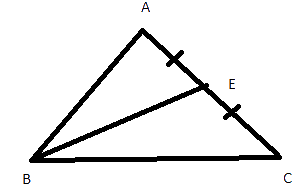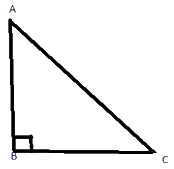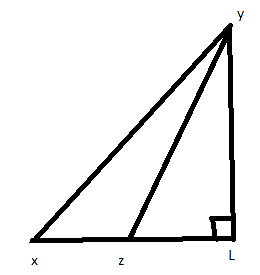# Ex.6.1 Q2 The Triangle and Its Properties - NCERT maths Class 7

Go back to  'Ex.6.1'

## Question

Draw rough sketches for the following:

(i) In $$∆ABC$$, $$BE$$  is a median.

(ii) In $$∆PQR$$, $$PQ$$ and $$PR$$ are altitudes of the triangle.

(iii) In $$∆XYZ$$, $$YL$$ is an altitude in the exterior of the triangle.

Video Solution
Triangle & Its Properties
Ex 6.1 | Question 2

## Text Solution

What is known?

Statement.

What is unknown?

Rough sketch for given statement.

Reasoning:

Read the statement carefully and draw a rough figure according to the statement.

Steps:

Here $$BE$$ is a median in triangle$$ABC$$ therefore $$AE = EC.$$Here $$PQ$$ and $$PR$$ are the altitudes of triangle $$PQR$$ and $$PQ ┴ PR$$.$$YL$$ is an altitude in the exterior of triangle $$XYZ$$Learn from the best math teachers and top your exams

• Live one on one classroom and doubt clearing
• Practice worksheets in and after class for conceptual clarity
• Personalized curriculum to keep up with school